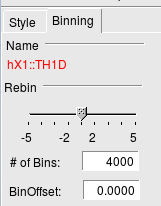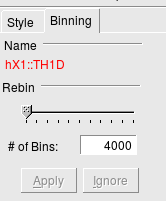# Rebinning histogram after axis calibration sometimes doesn't work

Hello,
(I will change “sometimes” in the title with the proper term, as soon as I learn it). Talking about rebinning, ROOT has two “kinds” of histograms. An image will explain best:That I will by now call “Type A”, and:“Type B”.

When I create an histogram, ROOT decides (I don’t know how: the decision changes e.g. changing the cut on the tree I project from) what “type” the histogram will be.
My problem is, if an 1D histogram is assigned “type A”, then, after I rescale the axis, for example like https://root-forum.cern.ch/t/can-we-shift-histogram-for-several-channels/12050/2 (I cannot do the `a->GetXbins()` thing because my histogram has “fix bins”), or to be short:

``````tree->Project("hist","e","t>4000");
TAxis* axis = hist->GetXaxis();
axis->Set(axis->GetNbins(), p0 + axis->GetXmin()*p1, p0 + axis->GetXmax()*p1 );
hist->ResetStats();
hist->Draw();
``````

If I now try to rebin the type A histogram, the bin contents sort of revert to before the axis rescaling. I mean: in the following, the big peak is at around 4100 keV and channel 2200. Here is the histogram after axis rescaling:

And this is what I see after clicking the bar for reducing the number of bins by 1/2:

If the histogram happens to be of type B, I instead get the expected result after rebinning.

If you want an example from me, I can give you my data and the cut you need to make ROOT decide for type A. But probably you know how to force the histogram to the type you want, so that we can test something very simple.
By the way, if you can tell me how to force degrading an histogram from type A to B (it does so by itself sometimes), that would be an acceptable workaround.

Thank you!

Can you provide a file with one histogram of each type ?

I tried today, and un(?)fortunately I found that, if I generate the type A histogram, then save it in a file (hist->Write(), or saving the whole canvas using the GUI), when I open it back it’s type B, and then the rebinning works (well, at least this means that saving the hist is a workaround).

So, I attach a tree with the two variables involved in the example in the first post root_forum_test.root (27.2 KB). It will suffice to open the tree, then:

``````Double_t p0=106,p1=1.8, EchMin=0.5,EchMax=4000.5;
TH1D* hX = new TH1D("hX","test", EchMax-EchMin,EchMin,EchMax);
TOF->Project("hX","e","t>4000"); //Change to e.g. Project("hX","e") to get type B
TAxis* axis = hX->GetXaxis();
axis->Set(axis->GetNbins(), p0 + axis->GetXmin()*p1, p0+ axis->GetXmax()*p1 );
hX->ResetStats();
hX->Draw();
``````

I get a Type A

with

``````TOF->Project("hX","e","t>4000");
``````

and with

``````TOF-> Project("hX","e") ;
``````

I do not understand what this slider with negative values means … May be you can doo the rebin not using the GUI ?

Oh? Interesting. It may be because I have only 6.10/08. In my few tests, I get type B every time I do not cut on a different variable than the one I plot.
Well, anyway, the problem happens exactly with type A, for me. Do you see the problem after changing the axis?

Under some obscure circumstances (i.e. when type A comes out), ROOT is able not only to reduce the number of bins, but also increase it, even beyond the number of bins the histogram was defined with: it’s controlled by that slider ("-2" = half the bins, “+5” = 5 times the bins). I guess it remembers the tree used for generating the histogram, so maybe it’s able do redo the bin assigments under the hood.
But also type B rebinning from GUI is quite useful: it can’t make more bins than there were originally, but after I rebin, say, from 4000 to 2000, I can go back to 4000 if I want, without creating the histogram again (like it is storing the original histogram in case I want it back).

Never tried before, let’s see. Yes, TH1::Rebin() makes the rebinning correctly, even after axis calibration. The histogram is still type A if I do hX->Rebin(2), and then if I now try to use the type A slider, it gives the same problem as before. If instead I create a new histogram hX->Rebin(2,“h2”), h2 is type B. So `hX->Rebin(1,"h2")` is a fast method to force a type B, by the way.

If you are happy with the `Rebin` method from a script that may solve your issue.